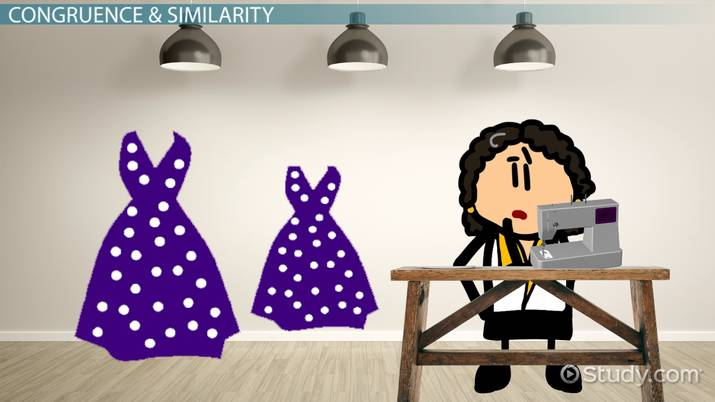# Practice Proving Relationships using Congruence & Similarity

Lesson Transcript
Instructor: Betsy Chesnutt

Betsy has a Ph.D. in biomedical engineering from the University of Memphis, M.S. from the University of Virginia, and B.S. from Mississippi State University. She has over 10 years of experience developing STEM curriculum and teaching physics, engineering, and biology.

In geometry, proving relationships using congruency and similarity can be done using different methods. Take a closer look at congruency and similarity, explore congruent triangles, and compare similar triangles. Updated: 11/11/2021

## Congruence and Similarity

Johanna likes to sew, and she decides to make some dresses for her friends. One friend is really small and one is very tall, so she can't use the same pattern for both dresses. She buys two different sizes of patterns for the dress and when she looks at the finished dresses side by side, she sees something very interesting.

The two dresses are exactly the same shape, but one is bigger than the other. Although Johanna may not have realized it, her observation about the similarity of the dress shapes she made reveals a real geometrical relationship between the two dresses. In geometry, if two figures are similar, they have the same shape but are different sizes, just like these two dresses.

In addition to being similar, two figures can also be congruent, but not all similar shapes are congruent, too. For shapes to be congruent as well as similar, they must have not only the same shape, but also the same size. If Johanna made multiple dresses that were all size small, then we would say that all those dresses were congruent.An error occurred trying to load this video.

Try refreshing the page, or contact customer support.

Coming up next: The AAS (Angle-Angle-Side) Theorem: Proof and Examples

### You're on a roll. Keep up the good work!

Replay
Your next lesson will play in 10 seconds
• 0:00 Congruence & Similarity
• 1:13 Congruent Triangles
• 3:23 Similar Triangles
• 4:31 Lesson Summary
Save Save

Want to watch this again later?

Timeline
Autoplay
Autoplay
Speed Speed

## Congruent Triangles

While we can look at the dresses and see that they are similar, this doesn't constitute mathematical proof. So, how can you prove that two shapes are congruent or similar? Let's use a triangle, a common geometrical shape, to illustrate the relationships between similar and congruent shapes. First, we'll go over some ways to prove that two triangles are congruent. Remember, shapes that are congruent will be exactly the same size and shape.

There are several ways to prove that triangles are congruent. One way is to compare each of the three sides of a triangle, referred to as the side-side-side (SSS) proof. Two triangles are congruent if each side in the first triangle is congruent (equal) to a side in the second triangle.

Our second proof is referred to as side-angle-side (SAS). Two triangles are congruent if two sides in one triangle and the angle in between them are congruent to the same sides and angle in the second triangle.

With the angle angle side (AAS) proof, we see that two triangles are congruent if two angles and one side (one that is not between the angles) in the first triangle are congruent to the same angles and side in the second triangle.

We can also look at a side between two angles. With the angle side angle (ASA), we know that two triangles are congruent if two angles and the side between them are congruent to the same angles and included side in the second triangle.

If you can show that any of these are true, then you know that the triangles are congruent.

What about two triangles that have the same angle measurements? Are they congruent as well? They might be, but just knowing the angle measurements of the triangles is not enough to prove that the triangles are congruent. Similar triangles also have equal angle measurements, but the sides may be different lengths.

To unlock this lesson you must be a Study.com Member.

### Register to view this lesson

Are you a student or a teacher?